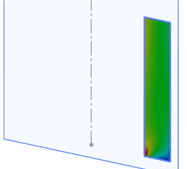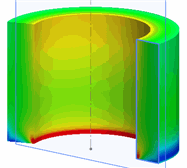# Viewing Results

After running a 2D simplification study, you can plot the results in 3D.

1. In the Simulation study tree, under the Results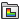folder, double-click an existing plot or create a new plot.
2. Right-click the plot and select Show as 3D Plot.
For plane stress, plane strain, and extruded simplification types, the results are extruded along the section depth. For the axisymmetric analysis type, the results are revolved by 330º about the axis of symmetry.

Alternatively, in the PropertyManager of a result plot, under Advanced Options, you can select Show as 3D plot.

For plane stress or plane strain assumptions, the results are the same for all cross sections parallel to the section plane.
 2D Results Plot 3D Results Plot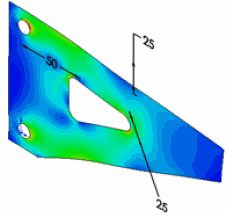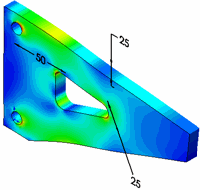For the axisymmetric assumption, the results are the same for all cross sections about the axis of revolution.
 2D Results Plot 3D Results Plot (rotated 200°)